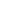# Free Pair of linear equations in two variable Worksheets

Download free printable Pair of linear equations in two variable Worksheets to practice. With thousands of questions available, you can generate as many Pair of linear equations in two variable Worksheets as you want.

## Sample Pair of linear equations in two variable Worksheet Questions

1.

if 2/x+3/y=10 and 3/x+2/y=5 ,then find 1/x + 1/y =........................

1.

3

2.

2

3.

4

4.

1/3

2.

Choose the correct option: The pair of equations x + 2y + 5 = 0 and –3x – 6y + 1 = 0 have _______.

1. A unique solution 2. Exactly two solutions 3. Infinitely many solutions 4. No solution
3.
A boatman rows his boat 35 km upstream and 55 km down stream in 12
hours. He can row 30 km. upstream and 44 km downstream in 10 hours.
Find the speed of he stream and that of the boat in still water. Hence find
the total time taken by the boat man to row 50 cm upstream and 77 km
downstream.
4.
In a function if 10 guests are sent from room A to B, the no. of guests
in room A and B are same. If 20 guests are sent from B to A, the no. of
guests in A is double the no. of guests in B. Find the number of guests in both.
the rooms in the beginning.
5.
In a function, Madhu wished to give Rs. 21 to each person present and
found that she fell short of Rs. 4 so she distributed Rs. 20 to each and
found that Rs. 1 were left over. How much money did she gave and how?
many persons were there.
6.
A mobile company charges a fixed amount as monthly rental which
includes 100 minutes free per month and charges a fixed amount there
after for every additional minute. Abhishek paid Rs. 433 for 370 minutes.
and Ashish paid Rs. 398 for 300 minutes. Find the bill amount under the
same plan, if Usha use for 400 minutes.
7.
Check graphically whether the pair of lines 3x + 2y – 4 = 0 and 2x – y
– 2 = 0 is consistent. Also find the coordinates of the points where the
graphs of the lines of equations meet the y-axis.
8.
For what values of k,the following equations have infinite solution Kx + 3y - (K-3) = 0 & 12x + Ky - K = 0
9.
Solve for x,y : 5/y - 2/x = 11 divided by 6 & 36/x - 24/y = 1
10.
Determine the value of K for which the given system of linear equations
has infinitely many solutions: Kx - 3y = K - 3 & 12x + Ky = K

Worksheets by UrbanPro

Our worksheets are designed to help students explore various topics, practice skills and enrich their subject knowledge, to improve their academic performance. Designed by Experts who have extensive experience and expertise in teaching a subject, these worksheets will improve your child's problem-solving skills and subject knowledge in a fun and interactive manner.
Check out our free customized worksheets across school boards, grades, subjects and levels of subject knowledge. You can download, print and share these worksheets with anyone, anywhere, anytime!

Get a custom worksheet to practice!

Select your topic & see the magic.

subjectSelect Chapter(s)

Chapters & Subtopics